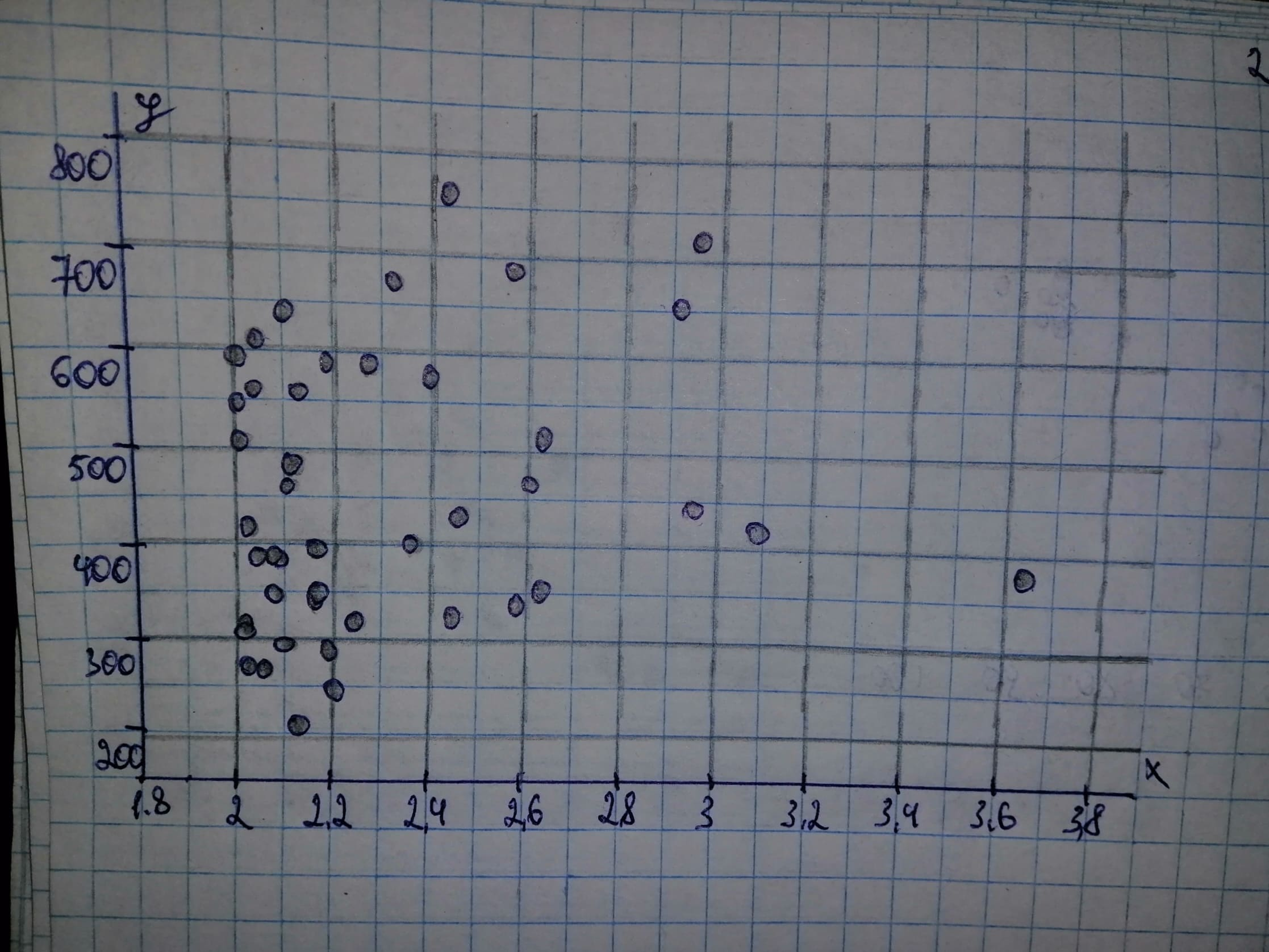# The document Arizona Residential Property Valuation System, published by the Arizona Department of Revenue, describes how county assessors use computeChardonnay Felix 2021-02-24 Answered
The document Arizona Residential Property Valuation System, published by the Arizona Department of Revenue, describes how county assessors use computerized systems to value single-family residential properties for property tax purposes. a) Obtain a scatterplot for the data. b) Decide whether finding a regression line for the data is reasonable. If so, then also do parts (c)-(f). c) Determine and interpret the regression equation for the data. d) Identify potential outliers and influential observations. e) In case a potential outlier is present, remove it and discuss the effect. f) In case a potential influential observation is present, remove it and discuss the effect.
You can still ask an expert for help

• Questions are typically answered in as fast as 30 minutes

Solve your problem for the price of one coffee

• Math expert for every subject
• Pay only if we can solve ityunitsiL

Given: a) Lot Size is on the horizontal axis and value is on the vertical axis.b) It is reasonable to find a regression lien for the data if there is no strong curvature present in the scatterplot. We note that there is no strong curvature in the scatterplot of part (a) and thus it is reasonable to find a regression line for the data. c) Let us first determine the necessary sums:

Next, we can determine ${S}_{xx}$ and ${S}_{xy}$

The estimate b of the slope $\beta$ is the ratio of ${S}_{xy}$ and ${S}_{xx}$: The mean is the sum of all values divided by the number of values:
The estimate a of the intercept $\alpha$ is the average of y decreased by the product of the estimate of the slope and the average of x. General least-squares equation: . Replace $\alpha$ by $a=292.0043$ and $\beta$ by $b=67.1128$ in the general least-squares equation: d) There appear to be no outliers, because the right most point lies much futher to the right than the other points in the graph. The outlier also appears to be a potential outlier, because it is possible that this point pulls the regression line down. e) Let us first determine the necessary sums:

Next, we can determine ${S}_{×}$ and